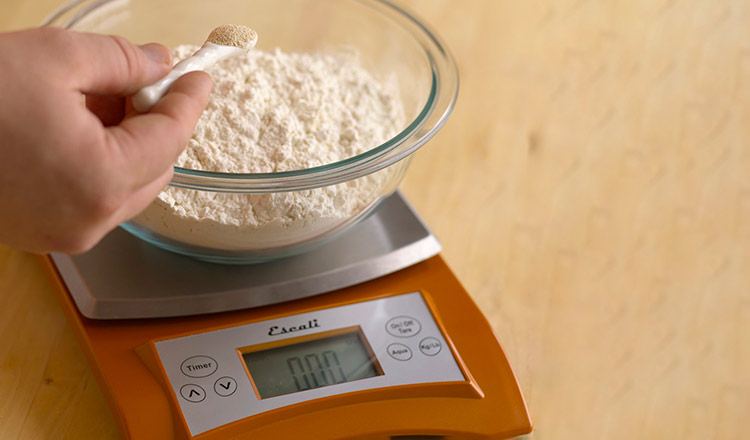Buckle up, foodies. We're doing math! Artisan bread requires just a few key ingredients, but it is the ratio of those ingredients and the way they are handled that produce a wide array of finished loaves. Proper execution comes from a detailed understanding of each step in the process—what needs to happen to produce a…

## CIA FOODIES

### Bakers’ Percentage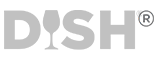### Buckle up, foodies. We're doing math!

Artisan bread requires just a few key ingredients, but it is the ratio of those ingredients and the way they are handled that produce a wide array of finished loaves. Proper execution comes from a detailed understanding of each step in the process—what needs to happen to produce a properly mixed dough, how to determine if a dough is properly proofed, the ideal oven conditions and temperature, and so on. The first step in any bread formula is scaling—that is, measuring ingredients for the perfect mix. Many home bakers rely on measuring cups to portion out their recipe ingredients by volume. It is probably what you were taught to do, and it may seem to work well enough. Professional bakers, however, are always looking for consistent results and they search for various ways to control the end product. Knowing that the amount of flour in a measuring cup can and does vary (due to humidity, settling, how and when the flour was milled, the type of cup being used and the way the cup was filled), professional bakers remove those variables by weighing their ingredients. Since they use scales to do this, it is called scaling. While many recipes are written in weight measures, bakers’ percentage is an accurate measurement used by professional bakers that presents ingredients as a percentage, rather than simply a weighable unit. The bakers’ percentage is used in all types of baking, but it is especially useful in bread baking. The concept is simple, an invaluable tool that helps bakers create new formulas and adjust current formulas. Used properly, bakers’ percentage can lead to consistency in production, better calculation of the absorption rate of the flour, and the ability to increase or decrease formula yields, as well as making it easier to compare formulas and offering a way to check a formula for balance and potential defects. The bakers’ percentage is always based on the total weight of the flour in the formula, which is always represented by the value of 100 percent. All other ingredients in the formula are calculated in relation to the flour, each assigned a percentage of its own based on the amount of it in the formula. If you are using half as much water as fl our in the recipe, then your water would be 50 percent, salt might be 2.3 percent, yeast might be 0.8 percent and so on. Because the flour is always 100 percent, your total percentage will add up to more than 100 percent. Once you have the percentages worked out, you can use them to scale a recipe up or down. Looking at the Bakers’ Percentages column in a recipe is also a good way to assess the type of bread you will be making. Some breads, such as brioche, will contain a high percentage of fat (an enriched dough) compared to a baguette, which has no added fats (a lean dough). Generally speaking, the bakers’ percentage is used only with weight measurements, not volume. All the ingredients are listed in similar units of measure. That is, all ingredients are listed in pounds or grams. Also, it is possible for ingredients to be more than 100 percent in the formula, a reflection of the dough in question. For example, some very hydrated doughs might have water listed at 125 percent. If the baker finds the dough to be overhydrated, he or she could alter the formula by adjusting the percentage of water.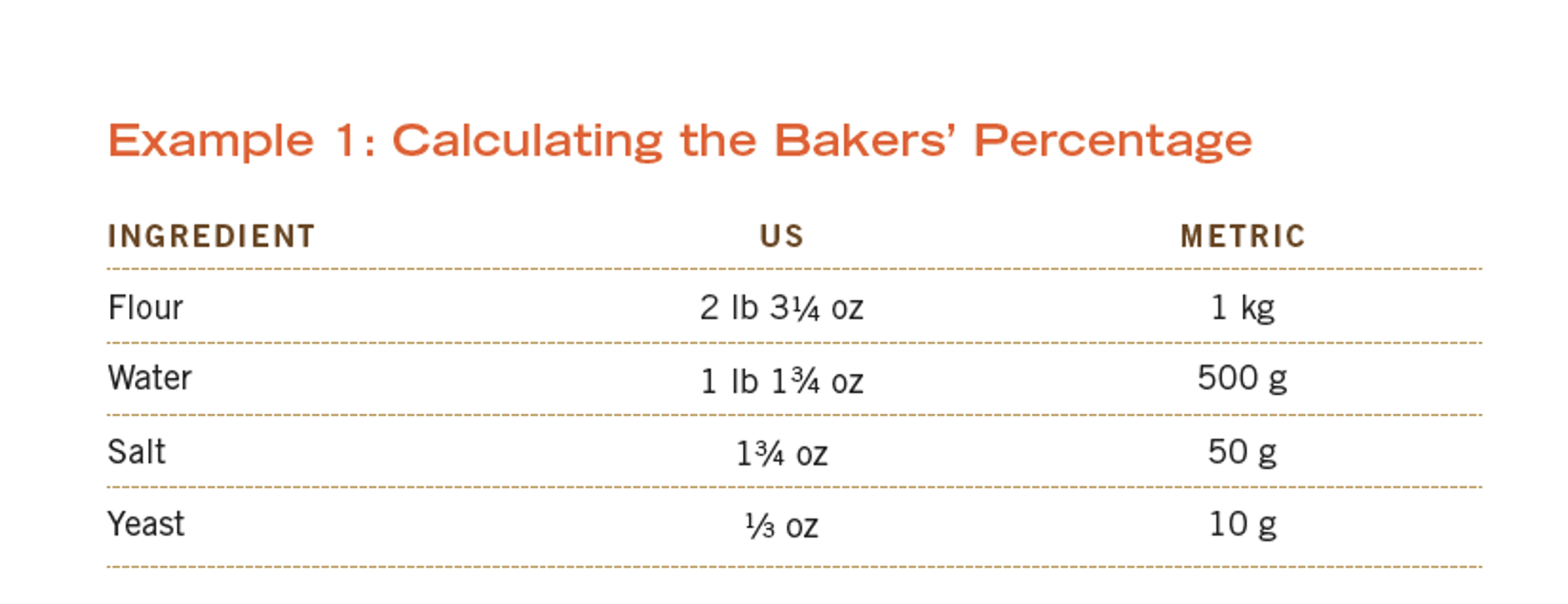As the base of the formula, the bakers’ percentage for the flour is 100 percent. The baker can then calculate the bakers’ percentage for the ingredients in one of the following ways:
Division: dividing the weight of the water by the weight of the flour gives the bakers’ percentage as 50 percent. For example,

#### = 0.50, or 50%

Cross Multiplication: multiplying the weight of the water by 100, then dividing by the weight of the flour gives the bakers’ percentage as 50 percent. For example,

#### = 0.50, or 50%

If the same calculations are applied to the entire formula, the completed recipe would read as follows: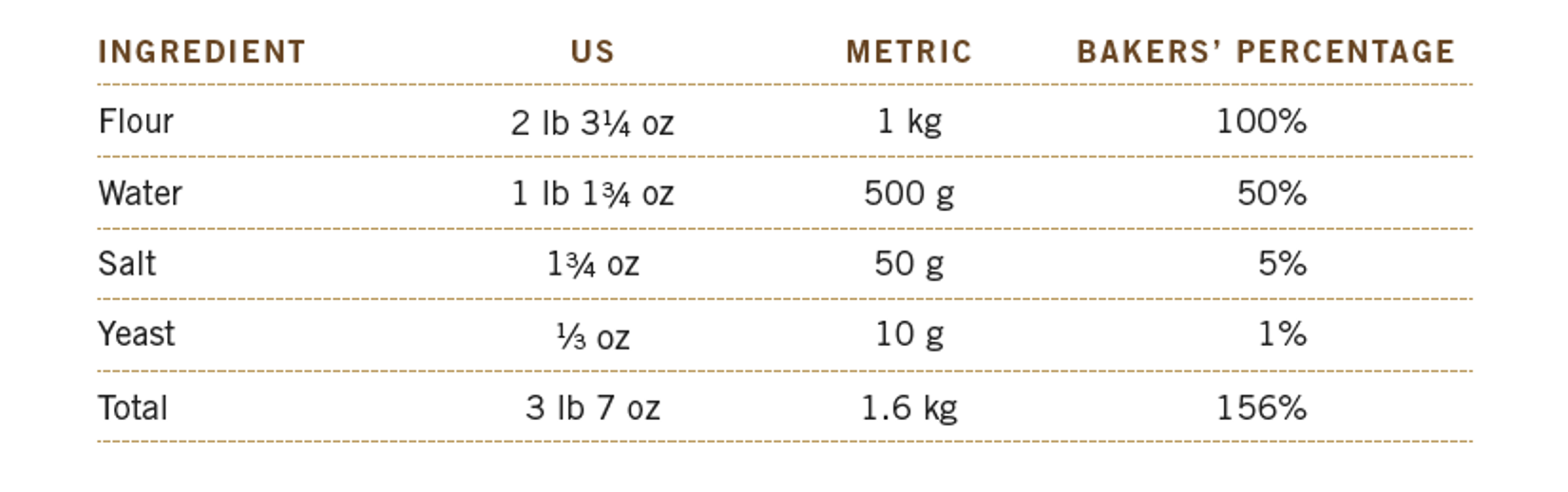The reverse method can also be used to determine the amounts for the ingredients in a formula, based on simply the percentages. This is especially useful for bakers looking to increase or decrease a formula to produce a specific yield. Say, for example, a baker wants to increase the example recipe to yield 18 lb 113 4 oz/8.5 kg dough. Here’s how it is done: Division: dividing the percentage for the flour (100%) by the total percentage for the full formula yields how much of the total formula is the flour. This number can then be multiplied by the desired yield to determine the correct flour amount.

#### = 12 lb1 4 oz/5.5 kg flour

Cross Multiplication: multiplying the percentage of the flour by the desired yield, then dividing by the total percentage of the formula yields the amount of flour needed in the recipe.

#### = 850,000 g/156 (total %) = 12 lb 1 4 oz/5.5 kg

If the same calculations are applied to the entire formula, the completed recipe would read as follows: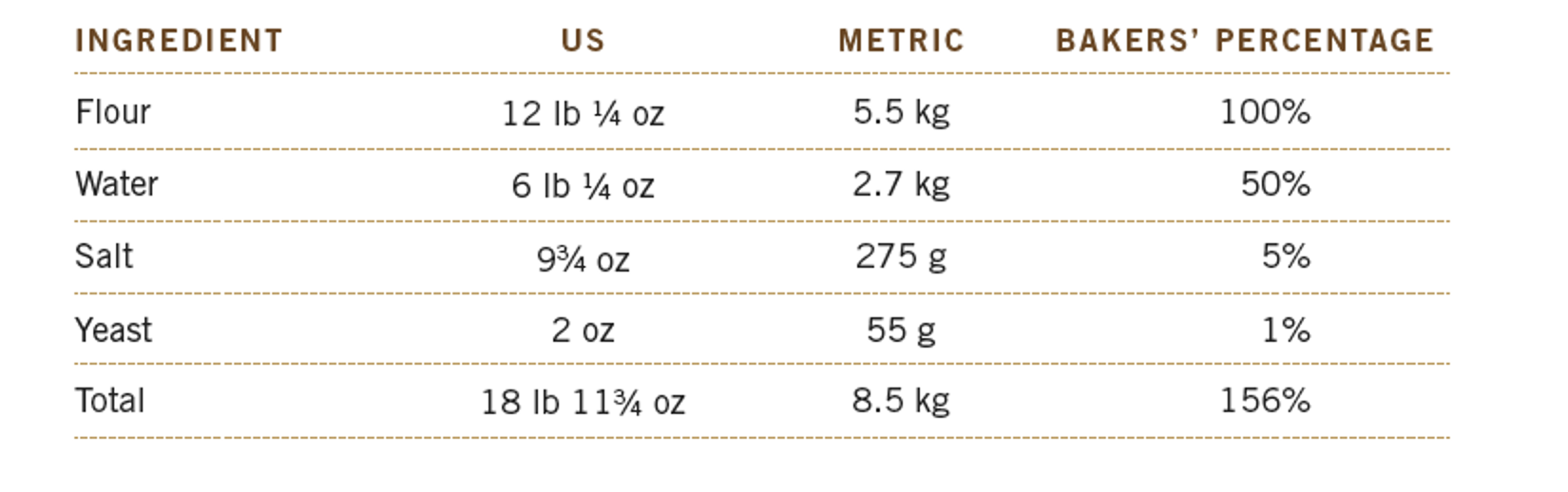1.2.